Logistic Regression: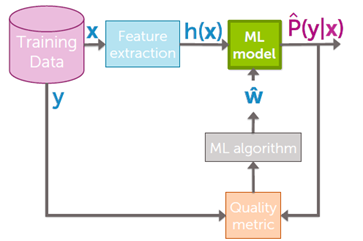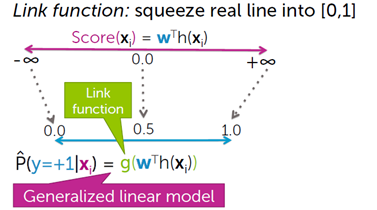Scores range from minus infinity to plus infinity, probabilities range between 0 and 1. The question is how do we relate score from minus infinity to plus infinity, to probability 0 and 1. How do we link these two things?

We are going to take the score, which is between minus infinity and plus infinity, we are going to push it through a function g that squeezes that huge line into the interval 0, 1. And uses it to predict the probability that y equals +1.

And when you're taking a linear model, w transpose h minus infinity to plus infinity and you're squeezing it into 0,1 using link functions you are building what's called a generalized linear model.

what's a generalized linear model?

It's just like a regression model, but you squeeze it into 0, 1 by pushing through a link function.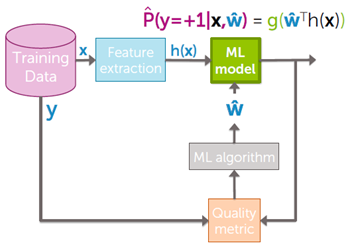The sigmoid (or logistic) link function:a. Classification and representation:

i. Classification:

To attempt classification, one method is to use linear regression and map all predictions greater than 0.5 as a 1 and all less than 0.5 as a 0. However, this method doesn't work well because classification is not actually a linear function.

The classification problem is just like the regression problem, except that the values y we now want to predict take on only a small number of discrete values. For now, we will focus on the binary classification problem in which y can take on only two values, 0 and 1. (Most of what we say here will also generalize to the multiple-class case.) For instance, if we are trying to build a spam classifier for email, x(i) then   may be some features of a piece of email, and y may be 1 if it is a piece of spam mail, and 0 otherwise. Hence, y∈ {0,1}. 0 is also called the negative class, and 1 the positive class, and they are sometimes also denoted by the symbols “-” and “+.” Given x(i), the corresponding y(i) is also called the label for the training example.

ii. Hypotheses representation:

We could approach the classification problem ignoring the fact that y is discrete-valued, and use our old linear regression algorithm to try to predict y given x. However, it is easy to construct examples where this method performs very poorly. Intuitively, it also doesn’t make sense for hθ(x) to take values larger than 1 or smaller than 0 when we know that y ∈ {0, 1}. To fix this, let’s change the form for our hypotheses hθ(x) to satisfy 0≤hθ(x)≤1. This is accomplished by plugging θTx  into the Logistic Function.

Our new form uses the "Sigmoid Function," also called the "Logistic Function":The following image shows us what the sigmoid function looks like: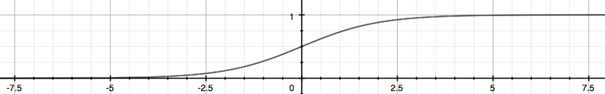The function g(z), shown here, maps any real number to the (0, 1) interval, making it useful for transforming an arbitrary-valued function into a function better suited for classification.

hθ(x) will give us the probability that our output is 1. For example, hθ(x)= 0.7 gives us a probability of 70% that our output is 1. Our probability that our prediction is 0 is just the complement of our probability that it is 1 (e.g. if probability that it is 1 is 70%, then the probability that it is 0 is 30%).iii. Decision Boundary:

In order to get our discrete 0 or 1 classification, we can translate the output of the hypothesis function as follows:The way our logistic function g behaves is that when its input is greater than or equal to zero, its output is greater than or equal to 0.5:Remember:So, if our input to g is x, then that means:From these statements we can now say:The decision boundary is the line that separates the area where y = 0 and where y = 1. It is created by our hypothesis function.

Example:In this case, our decision boundary is a straight vertical line placed on the graph where x1 = 5, and everything to the left of that denotes y = 1, while everything to the right denotes y = 0.

Again, the input to the sigmoid function g(z) (e.g. θTx) doesn't need to be linear and could be a function that describes a circle (e.g. z=θ01x122x22) or any shape to fit our data.

b. Logistic regression model:

i. Cost Function:

We cannot use the same cost function that we use for linear regression because the Logistic Function will cause the output to be wavy, causing many local optima. In other words, it will not be a convex function.

Instead, our cost function for logistic regression looks like:When y = 1, we get the following plot for J(θ) vs hθ(x):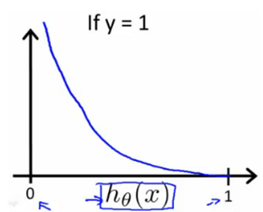Similarly, when y = 0, we get the following plot for J(θ) vs hθ(x):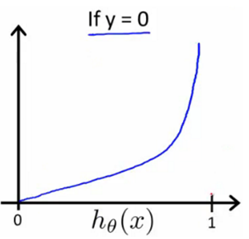If our correct answer 'y' is 0, then the cost function will be 0 if our hypothesis function also outputs 0. If our hypothesis approaches 1, then the cost function will approach infinity.

If our correct answer 'y' is 1, then the cost function will be 0 if our hypothesis function outputs 1. If our hypothesis approaches 0, then the cost function will approach infinity.

Note that writing the cost function in this way guarantees that J(θ) is convex for logistic regression.

ii. Simplified Cost Function and Gradient Descent:

We can compress our cost function's two conditional cases into one case:Notice that when y is equal to 1, then the second term (1-y)log(1-hθ(x)) will be zero and will not affect the result. If y is equal to 0, then the first term -ylog(hθ(x))will be zero and will not affect the result.

We can fully write out our entire cost function as follows:A vectorized implementation is:Remember that the general form of gradient descent is:

Repeat {}

We can work out the derivative part using calculus to get:

Repeat {}

Notice that this algorithm is identical to the one we used in linear regression. We still have to simultaneously update all values in theta.

A vectorized implementation is:"Conjugate gradient", "BFGS", and "L-BFGS" are more sophisticated, faster ways to optimize θ that can be used instead of gradient descent. We suggest that you should not write these more sophisticated algorithms yourself (unless you are an expert in numerical computing) but use the libraries instead, as they're already tested and highly optimized. Octave provides them.

We first need to provide a function that evaluates the following two functions for a given input value θ:We can write a single function that returns both of these: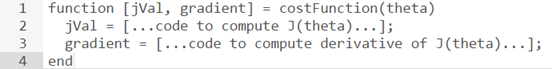Then we can use octave's "fminunc()" optimization algorithm along with the "optimset()" function that creates an object containing the options we want to send to "fminunc()". (Note: the value for MaxIter should be an integer, not a character string - errata in the video at 7:30)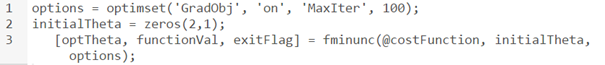We give to the function "fminunc()" our cost function, our initial vector of theta values, and the "options" object that we created beforehand.

c. Multiclass classification:

i. Multiclass Classification: One-vs-all :

Now we will approach the classification of data when we have more than two categories. Instead of y = {0,1} we will expand our definition so that y = {0,1...n}.

Since y = {0,1...n}, we divide our problem into n+1 (+1 because the index starts at 0) binary classification problems; in each one, we predict the probability that 'y' is a member of one of our classes.We are basically choosing one class and then lumping all the others into a single second class. We do this repeatedly, applying binary logistic regression to each case, and then use the hypothesis that returned the highest value as our prediction.

The following image shows how one could classify 3 classes: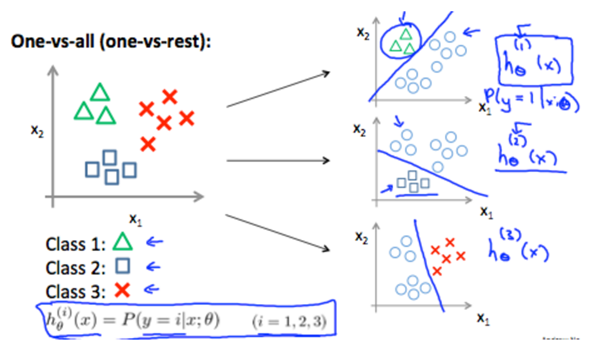To summarize:

Train a logistic regression classifier hθ(x) for each class to predict the probability that y = i.

To make a prediction on a new x, pick the class that maximizes hθ(x).

auhor: LASSRI Safae
PhD at faculty of science Ben M'Sik.